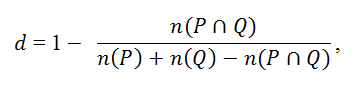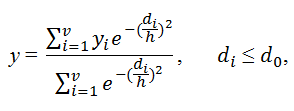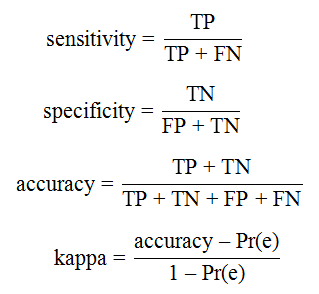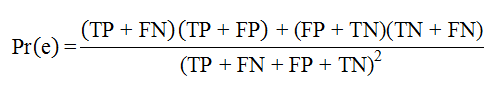#### The vNN Method

The vNN method rests on the premise that compounds with similar structures have similar activities. It is therefore reasonable to weight the contributions of neighbors so that closer neighbors contribute more to the predicted value. The vNN method calculates the similarity distance between molecules in terms of their structure, and uses a distance threshold to define a domain of applicability (i.e., all nearest neighbors that meet a minimum similarity threshold constraint). This applicability domain ensures that the predictions generated are reliable. vNN models can be built within minutes and require no re-training when new assay information becomes available—an important feature when keeping quantitative structure–activity relationship (QSAR) models up-to-date to maintain their performance levels. The performance characteristics of vNN-based models are comparable, and often superior to, those of other more elaborate model constructs.1,2,3,4
One of the most widely used measures of the similarity distance between two small molecules is the Tanimoto distance, d, which is defined as:where η(P∩Q) is the number of features common to molecules p and q, and η(P) and η(Q) are the total numbers of features for molecules p and q, respectively. The predicted biological activity y is then given by a weighted average across structurally similar neighbors:where di denotes the Tanimoto distance between a query molecule for which a prediction is made and a molecule i of the training set; d0 is a Tanimoto-distance threshold, beyond which two molecules are no longer considered to be sufficiently similar to be included in the average; yi is the experimentally measured activity of molecule i; v denotes the total number of molecules in the training set that satisfies the condition di≤d0; and h is a smoothing factor, which dampens the distance penalty. The values of h and d0 are determined from cross-validation studies. To identify structurally similar compounds, we used Accelrys extended-connectivity fingerprints with a diameter of four chemical bonds (ECFP4),5 which have previously been reported to show good overall performance.4,6,7

Model Validation
We use the 10-fold cross-validation (CV) procedure to validate new models and to determine the values of the smoothing factor h and Tanimoto distance d0. In this procedure, we randomly divided the data into 10 sets, and used 9 to develop the model and the 10th to validate it. We repeated this process 10 times, leaving each set of molecules out once. When building new models, we reported averages of the 10-fold CV as the performance measures.

Performance Measures
We use the following metrics to assess model performance. (1) sensitivity measures a model’s ability to correctly detect true positives, (2) specificity measures a model’s ability to detect true negatives, (3) accuracy measures a model’s ability to make correct predictions , and (4) kappa compares the probability of correct predictions to the probability of correct predictions by chance (its value ranges from +1 (perfect agreement between model prediction and experiment) to –1 (complete disagreement), with 0 indicating no agreement beyond that expected by chance).here TP, TN, FP, and FN denote the numbers of true positives, true negatives, false positives, and false negatives, respectively. Kappa is a metric for assessing the quality of binary classifiers. Pr⁡(e) is an estimate of the probability of a correct prediction by chance. It is calculated as:We also calculated the coverage, which is the proportion of test molecules with at least one nearest neighbor that meets the similarity criterion. The coverage is a measure of how many test compounds are within the applicability domain of a prediction model.

#### References

1. Liu, R., G. Tawa, and A. Wallqvist. Locally weighted learning methods for predicting dose-dependent toxicity with application to the human maximum recommended daily dose. Chemical Research in Toxicology. 2012; 25(10):2216-2226.
2. Liu, R., and A. Wallqvist. Merging applicability domains for in silico assessment of chemical mutagenicity. Journal of Chemical Information and Modeling. 2014; 54(3):793-800.
3. Liu, R., P. Schyman, and A. Wallqvist. Critically assessing the predictive power of QSAR models for human liver microsomal stability. Journal of Chemical Information and Modeling. 2015; 55(8):1566-1575.
4. Schyman, P., R. Liu, and A. Wallqvist. Using the variable-nearest neighbor method to identify P-glycoprotein substrates and inhibitors. ACS Omega. 2016; 1(5):923-929.
5. Rogers, D., and M. Hahn. Extended-connectivity fingerprints. Journal of Chemical Information and Modeling. 2010; 50(5):742-754.
6. Hert, J., P. Willett, D. Wilton, P. Acklin, K. Azzaoui, E. Jacoby, and A. Schuffenhauer. Comparison of topological descriptors for similarity-based virtual screening using multiple bioactive reference structures. Organic and Biomolecular Chemistry. 2004; 2:3256-3266.
7. Duan, J., S. Dixon, J. Lowrie, and W. Sherman. Analysis and comparison of 2D fingerprints: Insights into database screening performance using eight fingerprint methods. 2010; 29(2):157-170.

Contact us: v n n a d m e t @ b h s a i . o r g